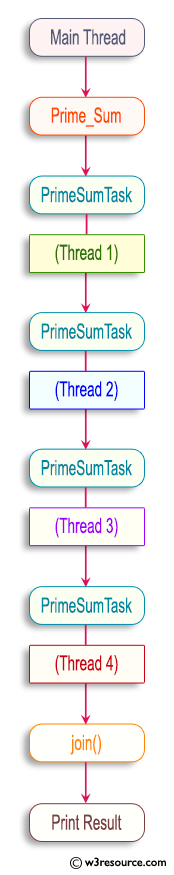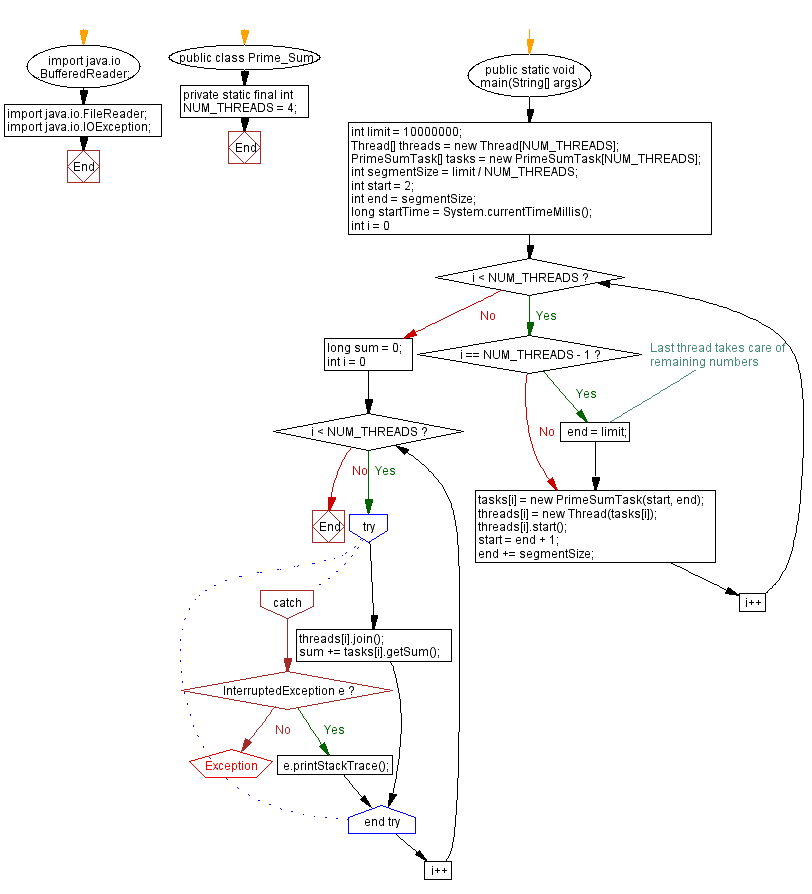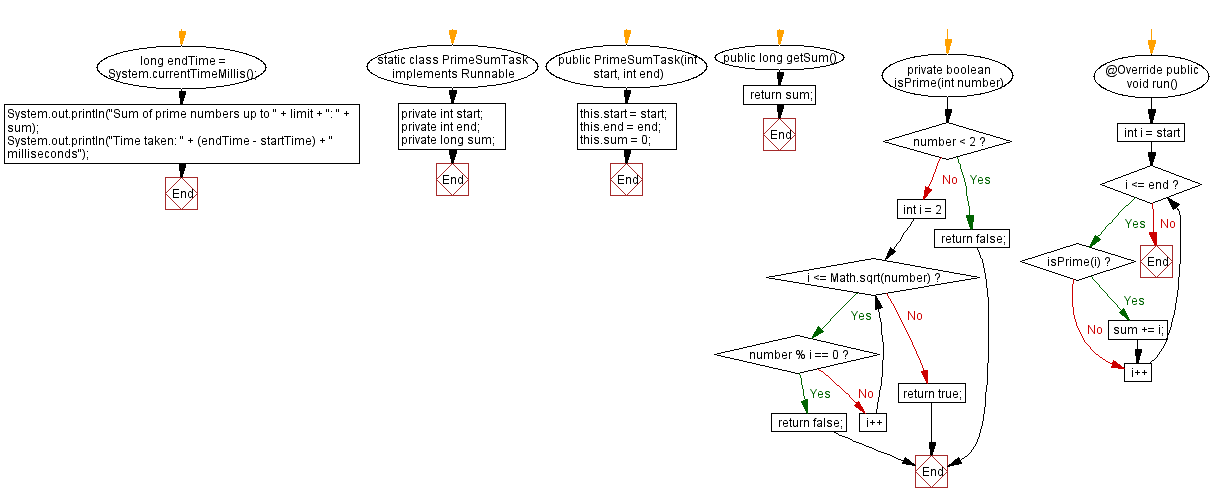﻿ Multithreaded Java Program: Sum of Prime Numbers

# Multithreaded Java Program: Sum of Prime Numbers

## Java Thread: Exercise-5 with Solution

Write a Java program that calculates the sum of all prime numbers up to a given limit using multiple threads.

Sample Solution:

Java Code:

``````import java.io.BufferedReader;
import java.io.IOException;

public class Prime_Sum {
private static final int NUM_THREADS = 4;

public static void main(String[] args) {
int limit = 10000000;

int segmentSize = limit / NUM_THREADS;
int start = 2;
int end = segmentSize;

long startTime = System.currentTimeMillis();

for (int i = 0; i < NUM_THREADS; i++) {
if (i == NUM_THREADS - 1) {
// Last thread takes care of remaining numbers
end = limit;
}

start = end + 1;
end += segmentSize;
}

long sum = 0;

for (int i = 0; i < NUM_THREADS; i++) {
try {
} catch (InterruptedException e) {
e.printStackTrace();
}
}

long endTime = System.currentTimeMillis();

System.out.println("Sum of prime numbers up to " + limit + ": " + sum);
System.out.println("Time taken: " + (endTime - startTime) + " milliseconds");
}

static class PrimeSumTask implements Runnable {
private int start;
private int end;
private long sum;

public PrimeSumTask(int start, int end) {
this.start = start;
this.end = end;
this.sum = 0;
}

public long getSum() {
return sum;
}

private boolean isPrime(int number) {
if (number < 2) {
return false;
}

for (int i = 2; i <= Math.sqrt(number); i++) {
if (number % i == 0) {
return false;
}
}

return true;
}

@Override
public void run() {
for (int i = start; i <= end; i++) {
if (isPrime(i)) {
sum += i;
}
}
}
}
}
```
```

Sample Output:

```Sum of prime numbers up to 10000000: 3203324994356
Time taken: 1800 milliseconds
```

Pictorial Presentation:Explanation:

In the above exercise,

• The Prime_Sum class is the main class of the program.
• The NUM_THREADS constant is set to 4, indicating the number of threads to be used for parallel processing.
• The main method is the program entry point. It starts by setting the limit variable, which determines the upper limit of the prime number range.
• The segmentSize variable is calculated by dividing the limit by the number of threads. This determines the size of each segment of numbers to be processed by each thread.
• Two variables, start and end, are initialized to specify the range of numbers to be processed by each thread. Initially, start is set to 2, which is the smallest prime number.
• Loops create and start threads. Each thread is assigned a segment of numbers to process. The last thread handles the remaining numbers that do not fit evenly into the segments. The PrimeSumTask class defines the thread's task.
• Inside the loop, PrimeSumTask objects are created with the corresponding start and end values. The threads are initialized with these tasks and started.
• After starting all the threads, a sum variable is initialized to hold the cumulative sum of prime numbers.
• Another loop waits for all threads to finish execution using the join() method. Within this loop, the sum of each task is accumulated in the sum variable.
• The program measures execution time by recording start and end times using System.currentTimeMillis().
• Finally, the total sum of prime numbers and the execution time are printed to the console.
• The PrimeSumTask class implements the Runnable interface and represents the thread's task. It takes the segment start and end values as parameters.
• The isPrime() method checks if a number is prime. It returns true if the number is prime, and false otherwise.
• The run() method defines the execution logic of each task. It iterates through each segment of numbers and checks if each number is prime. If a number is prime, it adds it to the sum variable.

Flowchart:Java Code Editor:

Improve this sample solution and post your code through Disqus

What is the difficulty level of this exercise?

Test your Programming skills with w3resource's quiz.

﻿

## Java: Tips of the Day

Converts a string from camelcase:

```public static String fromCamelCase(String input, String separator) {
return input
.replaceAll("([a-z\\d])([A-Z])", "\$1" + separator + "\$2")
.toLowerCase();
}
```

Ref: https://bit.ly/3u09A9E

We are closing our Disqus commenting system for some maintenanace issues. You may write to us at reach[at]yahoo[dot]com or visit us at Facebook